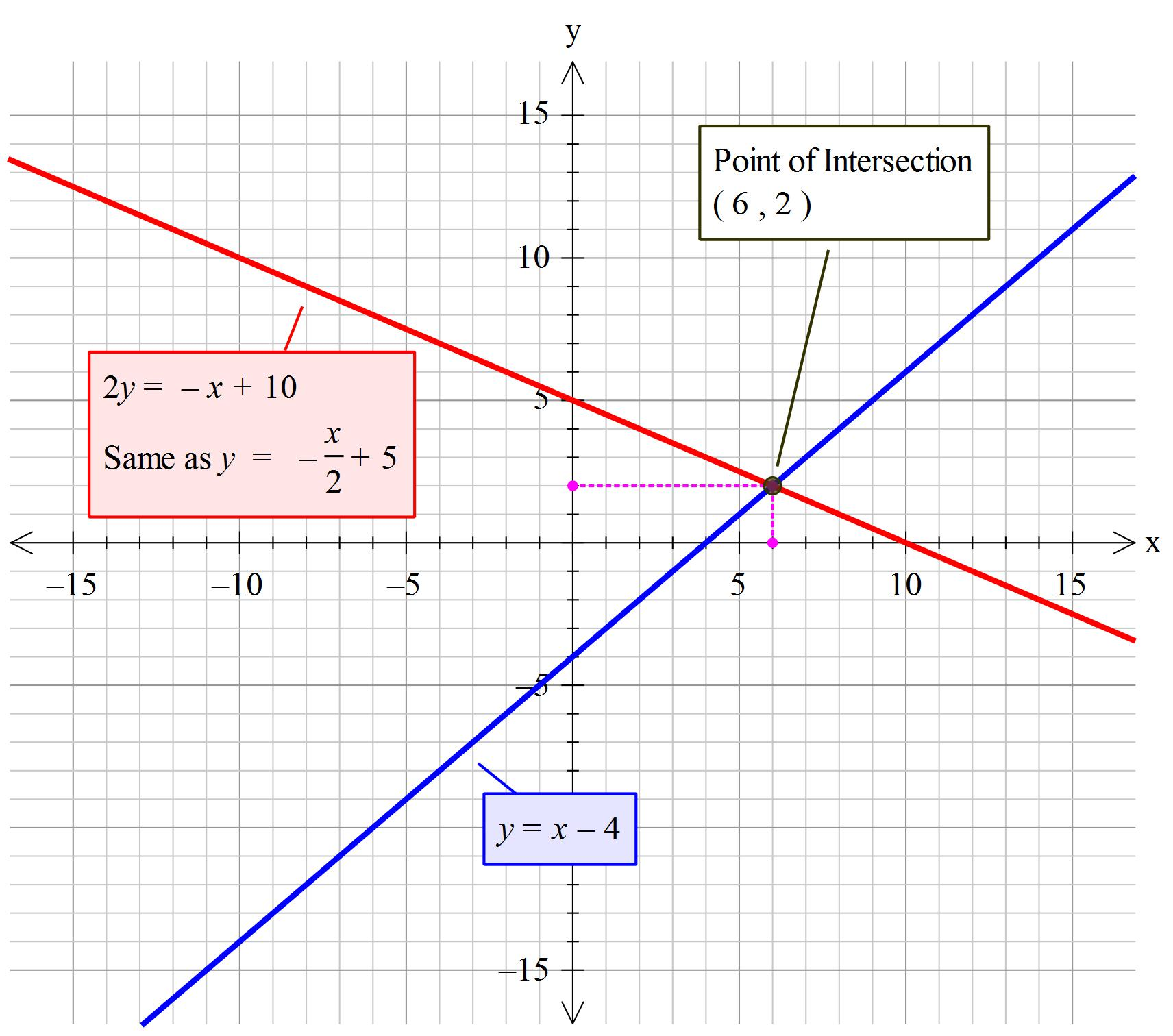Question

# How do you solve y=x-4, 2y=-x+10 by graphing and classify the system?

How do you solve y=x-4, 2y=-x+10 by graphing and classify the system?

Answer 1

The (shared points) point of intersection is at $\left(x , y\right) \to \left(6 , 2\right)$.

The system is of type: first order simultaneous equation system

#### Explanation:

For each equation:
Select at least 2 values for $x$ and substitute into the equations to determine the corresponding values of y. Draw a line through each point extending it to the edge of the squares on the graph paper.

$\textcolor{b l u e}{\text{Label all your points and put a title on your graph. Gives you extra}}$ $\textcolor{b l u e}{\text{marks for good communication.}}$

Equation 1
$y = x - 4$

Equation 2
$2 y = - x + 10 \text{ "color(red)(vec("divide both sides by 2"))" } y = - \frac{x}{2} + 5$
'~~~~~~~~~~~~~~~~~~~~~~~~~~~~~~~~~~~~~~~~~~~~~~~
The system is a first order simultaneous equation system

Note:
$x \to \text{first order}$
${x}^{2} \to \text{second order}$
${x}^{3} \to \text{third order}$Solution: The (shared points) point of intersection is at
$\left(x , y\right) \to \left(6 , 2\right)$. The system is a first order simultaneous equation system

#### Earn Coins

Coins can be redeemed for fabulous gifts.

Similar Homework Help Questions